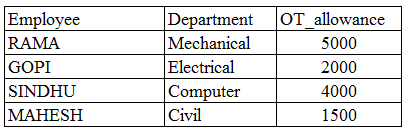# ISRO Scientist or Engineer Computer Science May 2017

Instructions

For the following questions answer them individually

Question 1

# If A is a skew symmetric matrix, then $$A^t$$Question 2

# If A and B be two arbitrary events, thenQuestion 3

# Using Newton-Raphson method, a root correct to 3 decimal places of the equation $$x^{3} - 3x - 5 = 0$$Question 4

# What does the data dictionary identify?Question 5

# Which of the following concurrency control protocol ensures both conflict serializability and free from deadlock?Question 6

# ACID properties of a transactions areQuestion 7

# Database table by name Overtime_allowance is given below :What is the output of the following SQL query? select count(*) from ((select Employee, Department from Overtime_allowance) as S natural join (select Department, OT_allowance from Overtime_allowance) as T);Question 8

# Which symbol denote derived attributes in ER Model?Question 9

# The symmetric difference of sets A = {1, 2, 3, 4, 5, 6, 7, 8} and B = {1, 3, 5, 6, 7, 8, 9} isQuestion 10

# The problems 3-SAT and 2-SAT areOR